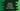# Python examples to create list of objects## Python example to create a list of objects :

Python list can hold a list of class objects. We can create one empty list and append multiple class objects to this list. Each list element will be an object, and we can access any member of that object like method, variables, etc. Note that you can append different class objects to the same list.

In this post, I will show you how to create one list of objects in python.

### Example 1: list of objects (same class instance) :

``````class Student:
def __init__(self, name, age):
self.name = name
self.age = age

studentList = []
studentList.append(Student("Alex", 20))
studentList.append(Student("Bob", 21))
studentList.append(Student("Ira", 15))

for student in studentList:
print('Name : {}, Age : {}'.format(student.name,student.age))``````

In this example, we are appending objects of the same type. Student is a class with two properties name and age. At first, we have initialized one empty list studentList and added three different Student objects to this list.

The for loop is used to print both properties of each object in that list.

It will print the below output :

``````Name : Alex, Age : 20
Name : Bob, Age : 21
Name : Ira, Age : 15``````

### Example 2: list of objects with different class instances :

``````class Student:
def __init__(self, name, age):
self.name = name
self.age = age

class Subject:
def __init__(self, name):
self.subjectName = name

data = []
data.append(Student("Alex", 20))
data.append(Subject("Subject-A"))
data.append(Student("Bob", 21))
data.append(Subject("Subject-B"))
data.append(Student("Ira", 15))

for item in data:
if(isinstance(item, Student)):
print('Name : {}, Age : {}'.format(item.name, item.age))
else:
print('Subject : {}'.format(item.subjectName))``````

In this example, we have two different classes Student and Subject. But we are appending objects of both of these classes to the same list data. The for loop is checking the type of the object before printing out its content.

It will produce the below output :

``````Name : Alex, Age : 20
Subject : Subject-A
Name : Bob, Age : 21
Subject : Subject-B
Name : Ira, Age : 15``````